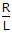# Electronics and Communication Engineering - Power Electronics

Exercise : Power Electronics - Section 6
1.
In a single phase full wave ac regulator feeding R-L load, the gate pulses
are always of short duration
may be of short duration
should be rectangular with width (p - a)
cannot be rectangular with width (p - a)
Explanation:
No answer description is available. Let's discuss.

2.
In a semi converter feeding a resistive load,
the waveshapes of input voltage and input current are always similar
the waveshapes of input voltage and input current can never be similar
the waveshapes of input voltage and input current are similar only if firing angle is zero
the waveshapes of input voltage and input current are similar only if firing angle is high
Explanation:
No answer description is available. Let's discuss.

3.
In a single phase semi-converter the number of thyristors is
16
8
4
2
Explanation:
No answer description is available. Let's discuss.

4.
A dc chopper is feeding an RLE load. The current can become discontinuous ifis high
a is low
E is high
none of the above